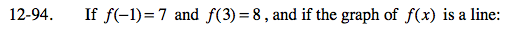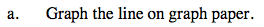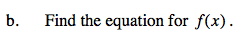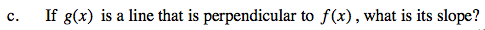### Home > CA > Chapter Ch12 > Lesson 12.4.3 > Problem12-94

12-94.
1. If f(−1) = 7 and f(3) = 8, and if the graph of f(x) is a line: Homework Help ✎

1. Graph the line on graph paper.

2. Find the equation for f(x).

3. If g(x) is a line that is perpendicular to f(x), what is its slope?f(-1) = 7 represents the point (-1, 7).
Graph this point and the point corresponding to f(3) = 8 in order to sketch the line.Use the y = mx + b format where m = slope (growth) and b = y-intercept (starting point).

Find the growth and substitute one of the coordinates of a point in the problem and solve for b.A line g(x) that is perpendicular to f(x) has a slope that is the negative reciprocal of the slope of the line f(x).